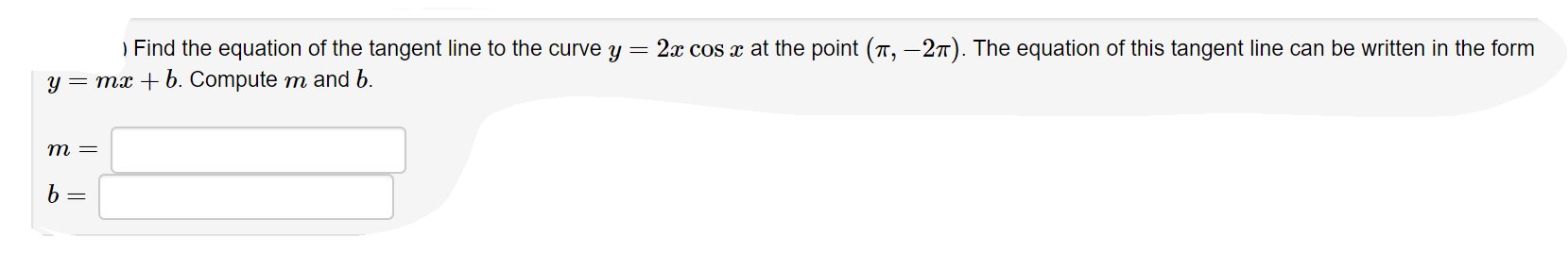# ) Find the equation of the tangent line to the curve y = 2x cos x at the point (π,-2π). The equation of this tangent line can be written in the formCompute m and

Questionhelp_outlineImage Transcriptionclose) Find the equation of the tangent line to the curve y = 2x cos x at the point (π,-2π). The equation of this tangent line can be written in the form Compute m and fullscreen
check_circleExpert Solution
Step 1

we have to find tangent to f(c) at x=pi

Step 2

now by the rule of derivative we find sl...

### Want to see the full answer?

See Solution

#### Want to see this answer and more?

Solutions are written by subject experts who are available 24/7. Questions are typically answered within 1 hour*

See Solution
*Response times may vary by subject and question
Tagged in

### Derivative